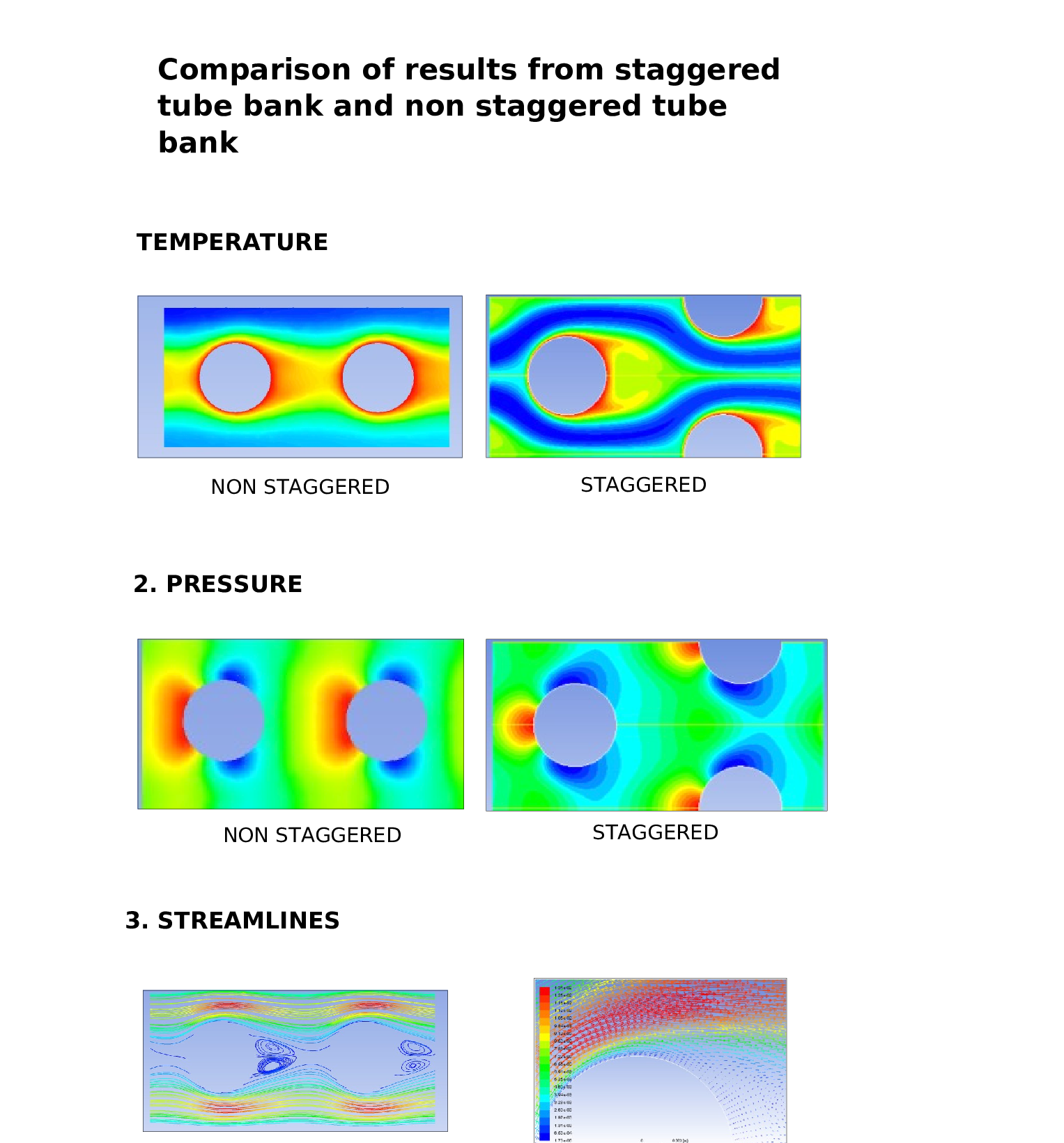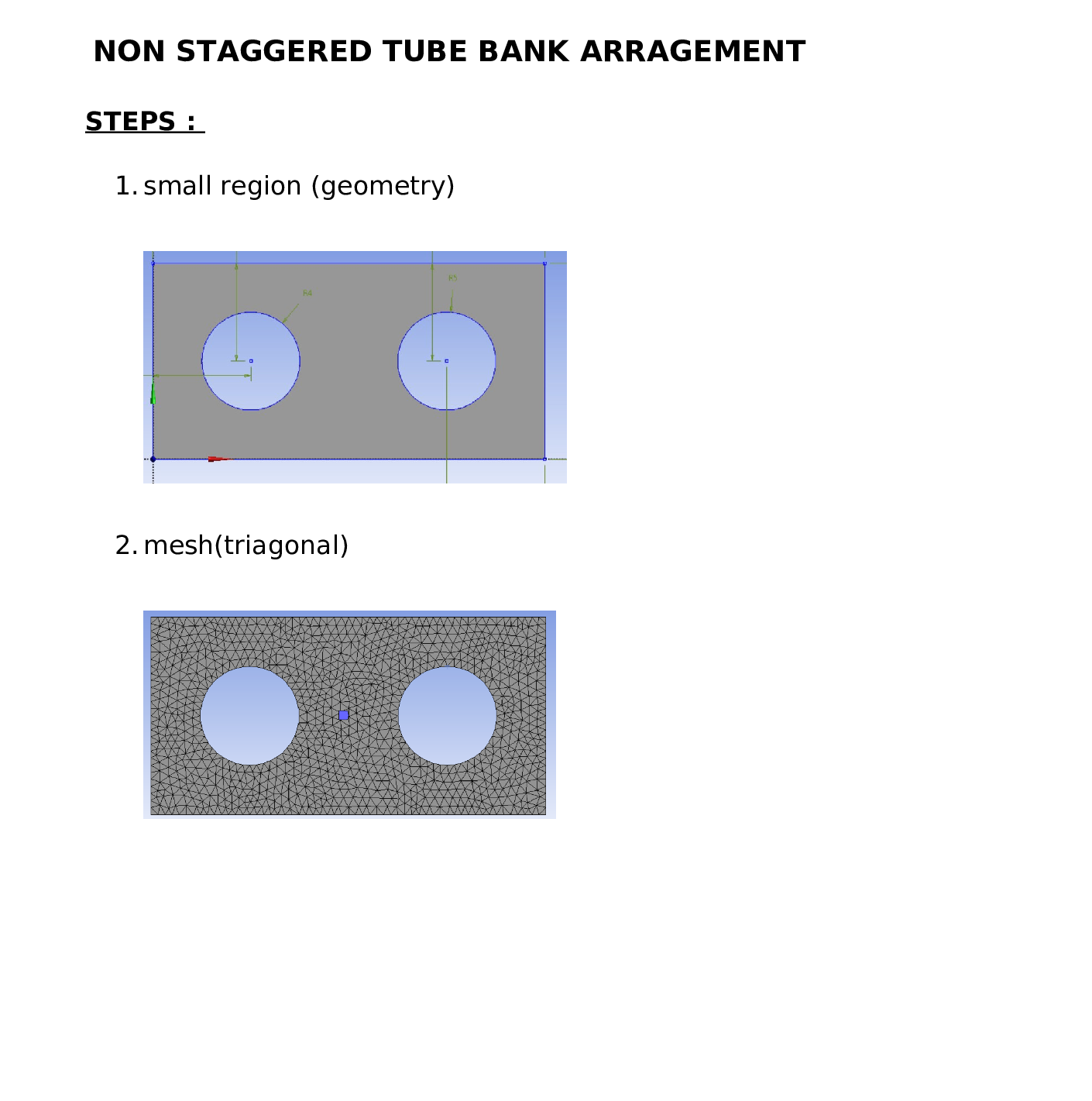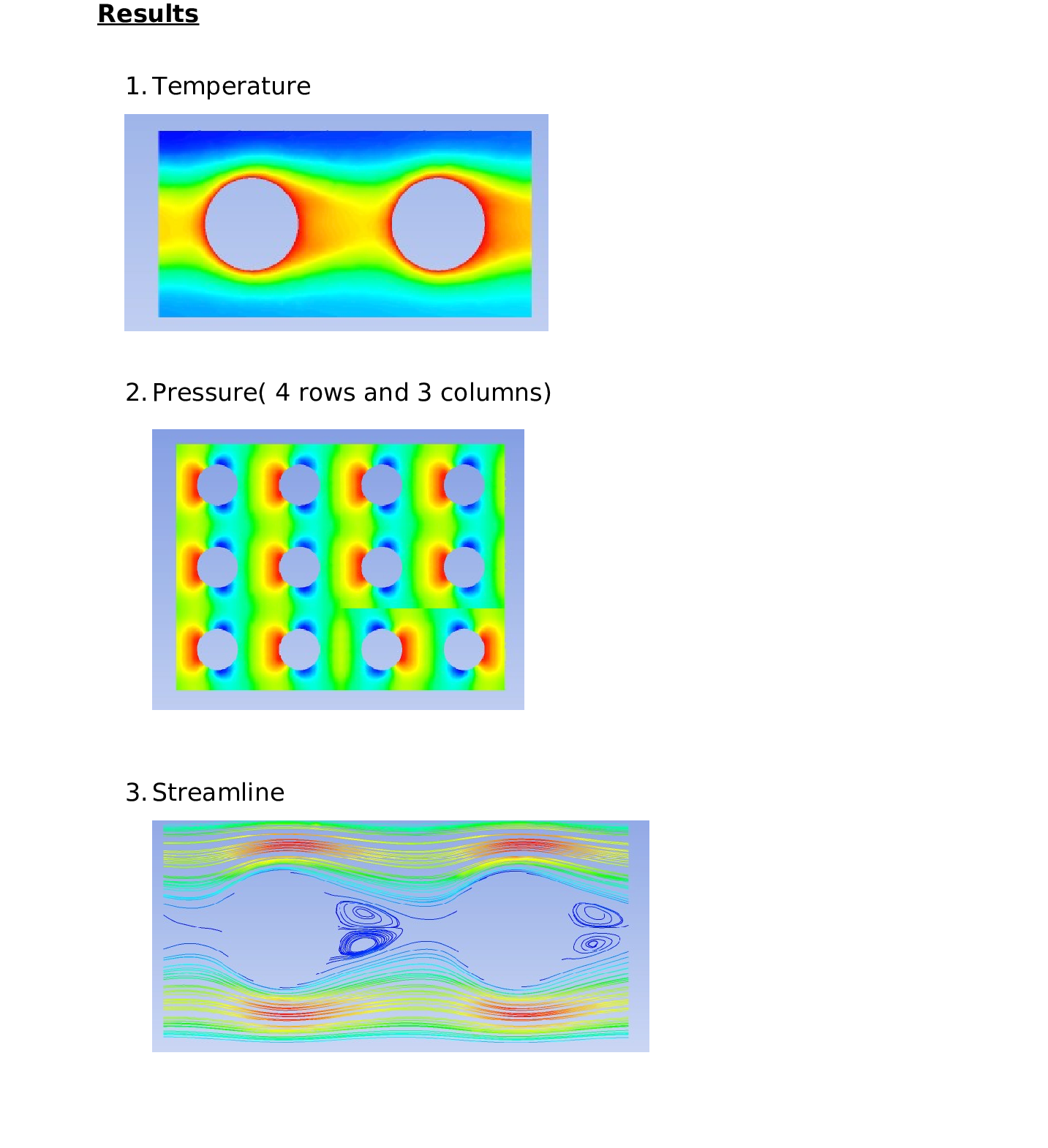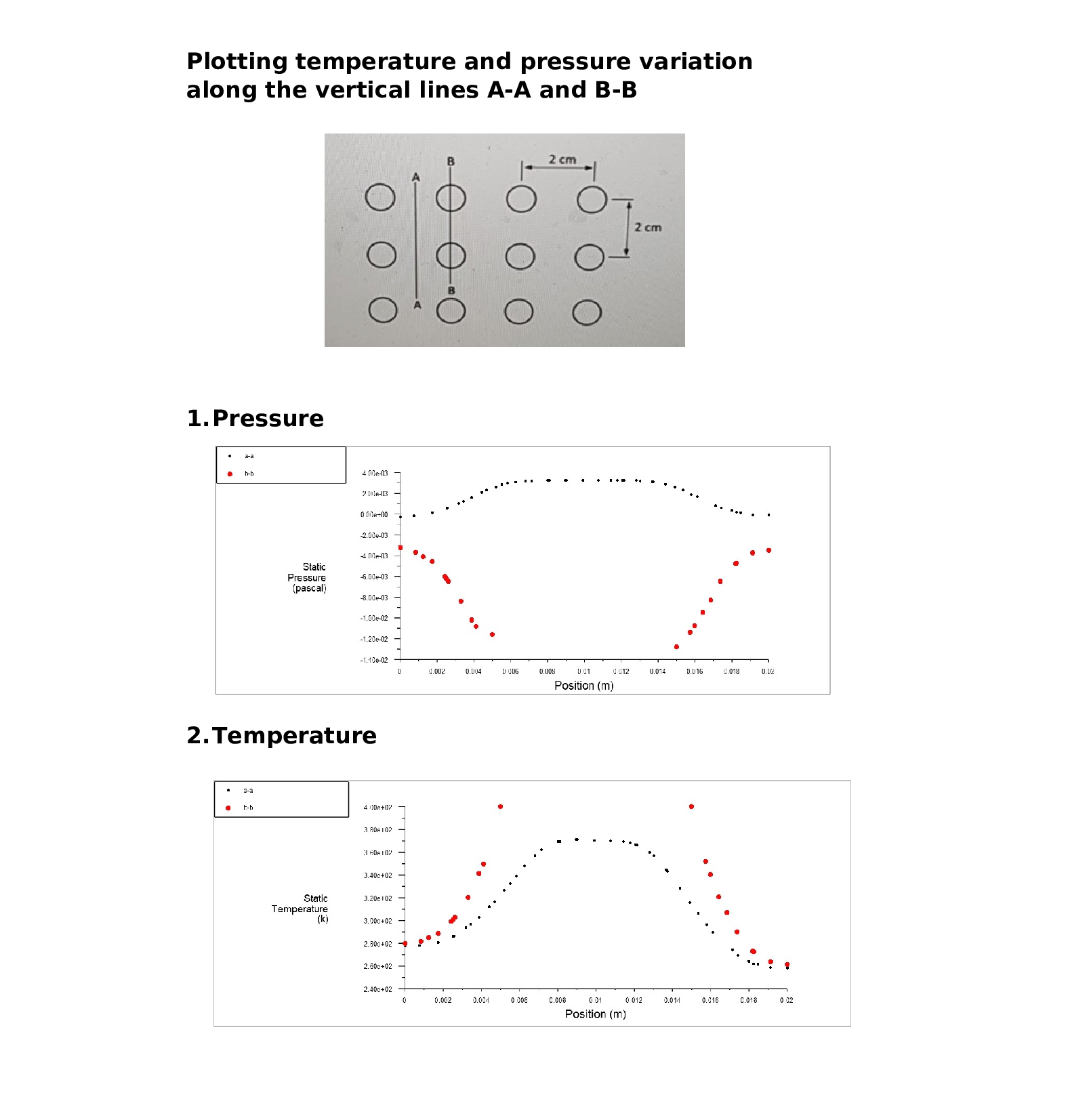Question

Computational Fluid Dynamics

Q2 Consider the non-staggered tube bank arrangement as shown below. All the conditions are the same as that from periodic tube bank heat transfer tutorial except the geometry. The tube diameter's 1cm,

You need: 1) identify a small region for the CFD simulation;

2) generate the appropriate geometry using ANSYS

3) generate the necessary mesh

4) follow the steps in the tutorial to perform CFD simulation of the heat transfer in a non-stagger tube bank

5) compare you results with that from the staggered tube bank and explain the difference in terms of heat transfer and pressure distribution around the cylinder.

6) show the pressure contours (show at least 3 rows and four columns of tube banks),

7) show the temperature contours

8) plot the streamlines

9) temperature and pressure variations along the vertical lines A-A and B-BVerified### Question 36302Computational Fluid Dynamics

Q2 Consider the non-staggered tube bank arrangement as shown below. All the conditions are the same as that from periodic tube bank heat transfer tutorial except the geometry. The tube diameter's 1cm,
You need: 1) identify a small region for the CFD simulation;
2) generate the appropriate geometry using ANSYS
3) generate the necessary mesh
4) follow the steps in the tutorial to perform CFD simulation of the heat transfer in a non-stagger tube bank
5) compare you results with that from the staggered tube bank and explain the difference in terms of heat transfer and pressure distribution around the cylinder.
6) show the pressure contours (show at least 3 rows and four columns of tube banks),
7) show the temperature contours
8) plot the streamlines
9) temperature and pressure variations along the vertical lines A-A and B-B

### Question 33862Computational Fluid Dynamics

RoR with interpolation

### Question 33861Computational Fluid Dynamics

(^Mone complex; involve F/P panytioning note baseline for reference

### Question 33860Computational Fluid Dynamics

17(Simple Conversion A/p

### Question 33859Computational Fluid Dynamics

Include CFD with each

### Question 13550Computational Fluid Dynamics

Q4: run the case of Re=5000 using transient solver. Monitor the lift force or lift coefficient of the cylinder. Estimate the oscillation frequency of the flow from the profile of life force/coefficient and compare it with the data in literature.
Experiments show that the frequency, f, of this periodic flow (in Hz) can be expressed in terms of a non-dimensional parameter known as the Strouhal number, defined as:
S t=\frac{f D}{U_{\infty}}

### Question 13549Computational Fluid Dynamics

Q3: Increase the inlet speed used in the previous question so that the Reynolds number becomes 5000, solve the problem again and provide new results. Before solving the problem initialize it,i.e. do not continue over the previous solution. Is there any unexpected behavior in this solution?If there is any, what is the cause of it? Provide the residual plot and discuss its behavior.

### Question 13548Computational Fluid Dynamics

Q2: Grid convergence is required for all CFD analysis. When grid size is reduced gradually, certain critical parameter gradually reaches convergence. In the present study, the drag coefficient can betaken as the critical parameter. Reduce the mesh size and solve the problem again, until grid convergence is achieved. Before solving the problem initialize it, i.e. do not continue over the previous solution.

### Question 13547Computational Fluid Dynamics

Consider a cylinder in the wind tunnel of the thermal fluid laboratory. The cylinder is about 10.5 inches long and has a diameter of 1.891 inch. The cross section of the duct is 10.5 inches wide and 15.75 inches high. The length of the test section is 42 inches and the cylinder is located approximately 16 inches downstream of the entrance to the test section. Now the three dimensional problem is simplified as two dimensional problem (Figure 1). The computational domain covers the whole duct.
Q1: the Reynolds number is defined as ReD=PUD/u where D is the diameter of the cylinder, U is the inlet velocity. Set up the case that has Reynolds number of 25. Make sure that the convergence tolerances are IE-5. Mesh size 10 mm with level 3 refinement around the cylindrical obstacle and on the wall. Initialize the problem and solve it. Provide the following results:
(a) Number of iterations needed for convergence.
(b) Drag coefficient
(c) Contour plot of velocity magnitude.
(d) Pathline plot
(e) x velocity profiles along the following five vertical lines: at the center of the cylinder (x=0),x= -50 mm, x= -26 mm, x = 50 mm, x= 400 mm, as approximately shown below.

### Question 2597Computational Fluid Dynamics

a)Describe why departure functions are used to calculate changes in thermodynamic properties(4)under non-ideal conditions.
(b)Butane gas undergoes a change of state from an initial condition of 2 MPa and 160°C to 3.5MPa and 227°C. Using departure functions and the thermodynamic data below calculate thechange in enthalpy and entropy.(12)
\text { The heat capacity at constant pressure is } c_{\mathrm{p}}=9.487+0.3313 T-1.108 \times 10^{-4} T^{2}-2.822 \times 10^{-9} T^{3} \text { in }
units of J kg1 K, where T is the temperature in kelvin. The enthalpic and entropicdeparture functions for butane are given by:
\text { At } 2 \mathrm{MPa} \text { and } 160^{\circ} \mathrm{C}, h-h^{\mathrm{ig}}=-2.4263 \mathrm{~kJ} / \mathrm{mol} \text { and } s-s^{\mathrm{i} 8}=-3.9507 \mathrm{~J} \mathrm{~mol}^{-1} \mathrm{~K}^{-1}
\text { At } 3.5 \mathrm{MPa} \text { and } 227^{\circ} \mathrm{C}, h-h^{i 8}=-3.2693 \mathrm{~kJ} / \mathrm{mol} \text { and } s-s^{\mathrm{i} 8}=-4.7567 \mathrm{~J} \mathrm{~mol}^{-1} \mathrm{~K}^{-1}
Using the Peng-Robinson equation of state, what is h- h for 56.11 g of 1-butene at 2 MPa and160°C?(c)(4)
You might find the PREOS spreadsheet useful to answering part (c), but you are welcome to useother sources of information if you wish. You must describe how you went out about answeringthis question, which is simply assessing your ability to obtain information.

### Submit query